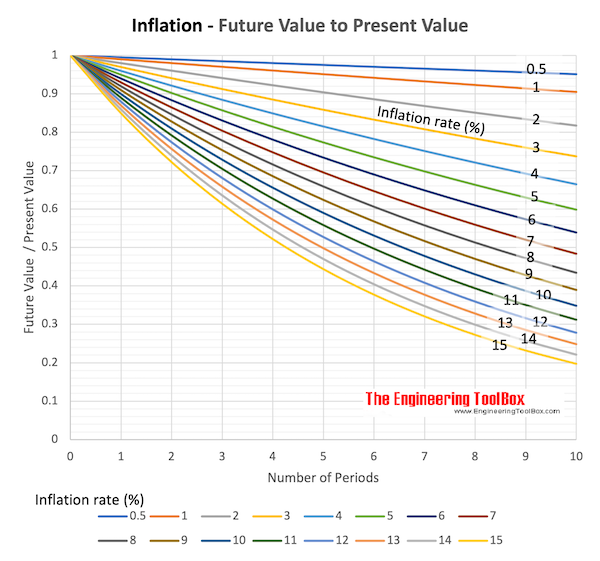Engineering ToolBox - Resources, Tools and Basic Information for Engineering and Design of Technical Applications!

# Inflation Rate

## Future value of money vs. inflation.

• An inflation with rising prices will decrease the value of money over time
• A deflation - the opposite of inflation or negative inflation - with decreasing prices will increase the value of money over time

### Inflation Rate

The future value of money after periods with uniform inflation rates can be expressed as

F = P (1 - i)n          (1)

where

F = future value

P = present value

i = average inflation (or deflation) rate per period (positive for inflation, negative for deflation)

n = number of periods

### Example - Inflation and Future Value

The future value of an amount of 100 after 10 periods and 4% of inflation rate can be calculated as

F = 100 (1 - 0.04)10 =

= 66.5

### Future Value of Present Money due to Inflation Rate - Calculator Table

This calculated table visualizes the decrease in future value of present money due to inflation rate and time.

###Download and print Inflation chart - Future Value vs. Present Value and Inflation Rate

### Variable Inflation Rate

The future value of money after periods with variable inflation rates can be calculated as

F = P (1 - i1) (1 - i2) ...... (1 - in)                  (2)

where

i1..n = inflation rates of terms

The average inflation rate for all periods can be calculated as

(1 - ia)n = (1 - i1) (1 - i2) ...... (1 - in)                (3)

or

ia = 1 - [(1 - i1) (1 - i2) ...... (1 - in)]1/n                    (3b)

where

ia = average inflation rate

## Related Topics

• ### Economics

Engineering economics - cash flow diagrams, present value, discount rates, internal rates of return - IRR, income taxes, inflation.

## Related Documents

• ### Contract Types

Commonly used engineering and construction contracts.
• ### Value Engineering

Improved value by enhanced function.

## Engineering ToolBox - SketchUp Extension - Online 3D modeling!

Add standard and customized parametric components - like flange beams, lumbers, piping, stairs and more - to your Sketchup model with the Engineering ToolBox - SketchUp Extension - enabled for use with older versions of the amazing SketchUp Make and the newer "up to date" SketchUp Pro . Add the Engineering ToolBox extension to your SketchUp Make/Pro from the Extension Warehouse !

We don't collect information from our users. More about

## Citation

• The Engineering ToolBox (2008). Inflation Rate. [online] Available at: https://www.engineeringtoolbox.com/inflation-rate-d_1236.html [Accessed Day Month Year].

Modify the access date according your visit.

9.27.11